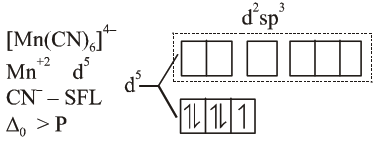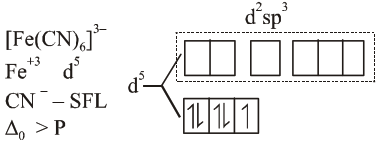# The hybridization and magnetic nature`
Question:

The hybridization and magnetic nature of $\left[\mathrm{Mn}(\mathrm{CN})_{6}\right]^{4}$ and $\left[\mathrm{Fe}(\mathrm{CN})_{6}\right]^{3-}$, respectively are:

1. $\mathrm{d}^{2} \mathrm{sp}^{3}$ and diamagnetic

2. $\mathrm{sp}^{3} \mathrm{~d}^{2}$ and diamagnetic

3. $\mathrm{d}^{2} \mathrm{sp}^{3}$ and paramagnetic

4. $\mathrm{sp}^{3} \mathrm{~d}^{2}$ and paramagnetic

Correct Option: , 3

Solution:$\therefore$ hybridisation is $\mathrm{d}^{2} \mathrm{sp}^{3}$ and due to presence of unpaired $e^{-}$complex is paramagnetic in nature$\therefore$ hybridisation is $\mathrm{d}^{2} \mathrm{sp}^{3}$ and due to presence of unpaired e complex paramagnetic in nature